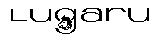Lugaru's Epsilon
Programmer's
EditorPrevious Up Next cygwin-root Variable Reference default-add-final-newline

### date-format: preference variable

Default value: `"%D-%t-%y %h:%N:%E %am"`

When the insert-date command inserts the current time and date, or Epsilon displays a time and date in certain other contexts, it formats the date using this variable. It can include any of the following sequences, and Epsilon will substitute the indicated value for that sequence:

%i
Epsilon substitutes the current hour in the range 0 to 23.

%h
Epsilon substitutes the current hour in the range 1 to 12.

%n
Epsilon substitutes the current minute in the range 0 to 59.

%e
Epsilon substitutes the current second in the range 0 to 59.

%a
Epsilon substitutes "a" before noon, "p" otherwise.

%d
Epsilon substitutes the day of the month in the range 1 to 31.

%m
Epsilon substitutes the month number in the range 1 to 12.

%f
Epsilon substitutes the English month name like January or February.

%t
Epsilon substitutes a 3-letter month abbreviation like Jan or Feb.

%y
Epsilon substitutes the four digit year.

%Y
Epsilon substitutes the two digit year.

%w
Epsilon substitutes the name of the day of the week, such as Friday.

%s
Epsilon substitutes the name of the day of the week, abbreviated to 3 characters, such as Fri.

%%
Epsilon substitutes a literal "%" character.

Using an uppercase letter instead of a lowercase letter for the sequences `%i`, `%h`, `%n`, `%e`, `%d`, or `%m` makes Epsilon always use two digits instead of one. For instance, at 3:07 pm, `%h%N` produces `3:07`, while `%H%n` produces `03:7`.

Using an uppercase letter instead of a lowercase letter for the sequences `%a`, `%f`, `%w`, `%s`, or `%t` makes Epsilon insert an uppercase version of the specified month, weekday, or AM/PM indicator.Copyright (C) 1984, 2020 by Lugaru Software Ltd. All rights reserved.